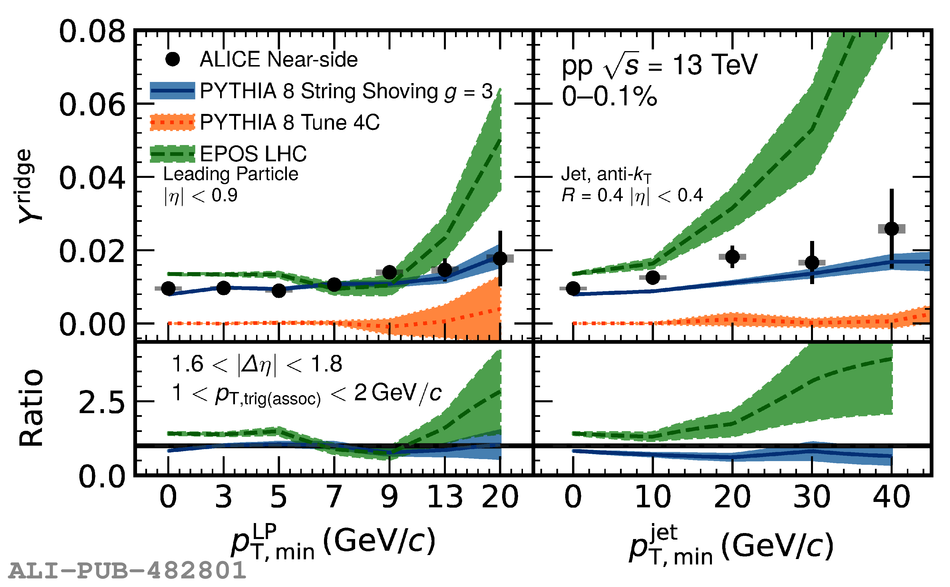# Figure 6

 < p>Near-side ridge yield as a function of the $\it{p}^{\rm{LP}}_{\rm{T,min}}$ (left) and $\it{p}^{\rm{Jet}}_{\rm{T,min}}$ (right). Data points (filled circles) show the ALICE measurement. The statistical and systematic uncertainties are shown as vertical bars and boxes, respectively. As the ridge yield is obtained in the same operational way for data and models, the upper limit of the systematic uncertainty due to jet contamination, which is 18.9\%, is not included in the figure. The data are compared with predictions of models which are represented by colored bands. The bottom panel shows a ratio of the models to the data. The uncertainty of the data is represented by the gray band centered around unity.< /p>